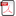### A Proof “P≠NP” for P vs. NP Problem by Multiple-Tape Turing-Machine

•  Yaozhi Jiang

#### Abstract

P vs. NP problem is very important research direction in computation complexity theory. In this paper author, by an engineer’s viewpoint, establishes universal multiple-tape Turing-machine and k-homogeneous multiple-tape Turing-machine, and by them we can obtain an unified mathematical model for algorithm-tree, from the unified model for algorithm-tree, we can conclude that computation complexity for serial processing NP problem if under parallel processing sometimes we can obtain P=NP  in time-complexity, but that will imply another NP, non-deterministic space-complexity NP, i.e., under serial processing P≠NP  in space-complexity, and the result is excluded the case of NP problem that there exists a faster algorithm to replace the brute-force algorithm, and hence we can proof that under parallel processing time-complexity is depended on space-complexity, and vice verse, within P vs. NP problem, this point is just the natural property of P vs. NP problem so that “P≠NP ”.

• Full Text:PDF
• DOI:10.5539/jmr.v12n4p1This work is licensed under a Creative Commons Attribution 4.0 License.
• ISSN(Print): 1916-9795
• ISSN(Online): 1916-9809
• Started: 2009
• Frequency: bimonthly

### Journal Metrics

• h-index (February 2019): 18
• i10-index (February 2019): 48
• h5-index (February 2019): 7
• h5-median (February 2019): 10